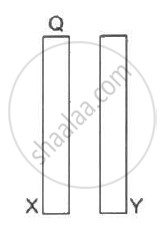Department of Pre-University Education, Karnataka course PUC Karnataka Science Class 12
Share

# Two Conducting Plates X and Y, Each with a Large Surface Area a (On One Side), Are Placed Parallel to Each Other, as Shown in the Following Figure. - Physics

ConceptElectric Field Introduction of Electric Field

#### Question

Two conducting plates X and Y, each with a large surface area A (on one side), are placed parallel to each other, as shown in the  following figure . Plate X is given a charge Q,whereas the other is kept neutral. Find (a) the surface charge density at the inner surface of plate X (b) the electric field at a point to the left of the plates (c) the electric field at a point in between the plates and (d) the electric field at a point to the right of the plates.#### Solution

(a)
Given that the charge present on the plate is Q. The other plate will get the same charge Q due to convection.

Let the surface charge densities on both sides of the plate be σ1 and σ2.

Now, electric field due to a plate,

"E" = sigma/(2∈_0)

So, the magnitudes of the electric fields due to this plate on each side

= sigma_1 /(2∈_0) and sigma_2/(2∈_0)

The plate has two sides, each of area A. So, the net charge given to the plate will be equally distributed on both the sides.This implies that the charge developed on each side will be

"q"_1 = "q"_2 = "Q"/2

This implies that the net surface charge density on each side = "Q"/(2"A")

(b)
Electric field to the left of the plates

On the left side of the plate surface, charge density,

sigma ="Q"/(2"A")

Hence, electric field ="Q"/(2"A"∈_0)

This must be directed towards the left, as 'X' is the positively-charged plate.

(c) Here, the charged plate 'X' acts as the only source of electric field, with positive in the inner side. Plate Y is neutral. So, a negative charge will be induced on its inner side. 'Y' attracts the charged particle towards itself. So, the middle portion E is towards the right and is equal to

"Q"/(2"A"∈_0)

d) Similarly for the extreme right, the outer side of plate 'Y'  acts as positive and hence it repels to the right with "E" = "Q"/(2"A"∈_0)

Is there an error in this question or solution?

#### APPEARS IN

Solution Two Conducting Plates X and Y, Each with a Large Surface Area a (On One Side), Are Placed Parallel to Each Other, as Shown in the Following Figure. Concept: Electric Field - Introduction of Electric Field.
S